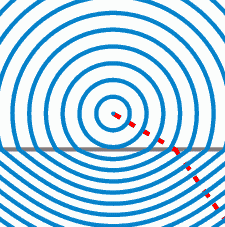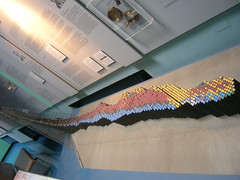Archive | September, 2012

## Waves,Properties and Their Applications.

4 Sep
1.316/365 Revision Week: Partial Differential Equations (Photo credit: stuartpilbrow)English: Propagation of a plane compression wave (impulse); made with Scilab and Jasc Animation Shop 2.02 Deutsch: Vorstellung einer Longitudinalwelle (impuls); gemacht mit Scilab und Jasc Animation Shop 2.02 Français : Propagation d’une onde de compression plane (impulsion) ; créé avec Scilab et Jasc Animation Shop 2.02 (Photo credit: Wikipedia)Refractive Indices (Photo credit: the_himay)English: Illustration of wavefronts in the context of Snell’s law. (Photo credit: Wikipedia)

Understand and use the terms amplitude, frequency, period, speed and wavelength

1. Identify the different regions of the electromagnetic spectrum and describe some of their applications.
1. Use the wave equation v = fλ.
1. Recall that a sound wave is a longitudinal wave which can be described in terms of the displacement of molecules.
1. Use graphs to represent transverse and longitudinal waves, including standing waves.
1. Explain and use the concepts of wavefront, coherence, path difference, superposition and phase.
1. Recognize and use the relationship between phase difference and path difference.
1. Explain what is meant by a standing (stationary) wave, investigate how such wave is formed, and identify nodes and antinodes.
1. Recognize and use the expression for refractive index 1μ2  = sin i/sin r = v1/v2 determine refractive index for a material in the laboratory, and predict whether total internal reflection will occur at an interface using critical angle.
1. Investigate and explain how to measure refractive index.
1. Investigate and explain how to measure the rotation of the plane of polarization.
1. Investigate and recall that waves can be diffracted and that substantial diffraction occurs when the size of the gap or obstacle is similar to the wavelength of the wave.
1. Explain how diffraction experiments provide evidence for the wave nature of elections.
1. Discuss how scientific ideas may change over time, for example, our ideas on the particle/wave nature of electrons.
1. Recall that, in general, waves are transmitted and reflected at an interface between media.
1. Explain how different media affect the transmission/reflection of waves travelling from one medium to another.
1. Explore and explain how a pulse-echo technique can provide details of the position and/or speed of an object and describe applications that use this technique.
1. Explain qualitatively how the movement of a source of sound or light relative to an observer/detector gives rise to a shift in frequency (Doppler Effect) and explore applications that use this effect.
1. Explain how the amount of detail in a scan may be limited by the wavelength of the radiations or by the duration of the pulses.
1. Discuss the social and ethical issues that need to be considered, e.g., when developing and trailing new medical techniques on patients or when funding a space mission.
Aside

## Astrophysics and Cosmology

2 SepIntended as a replacement for File:Universe.svg and File:Universos.gif. Improvements: better dash patterns, more accurate curves (actual solutions of the Friedmann equations, not hand-drawn). (Photo credit: Wikipedia)Curve of Binding Energy Gamowtal Deutsches Museum (Photo credit: brewbooks)The path of a star with the mass of the Sun over Hertzsprung-Russell diagram during stellar evolution. (Photo credit: Wikipedia)The Big Crunch. The vertical axis can be considered as either plus or minus time. (Photo credit: Wikipedia)English: Graph of a function of total emitted energy of a black body j^{\star} proportional to the fourth power of its thermodynamic temeprature T , according to the Stefan–Boltzmann law. In blue is a total energy according to the Wien approximation, equals j^{\star} / \zeta(4) \approx 0.923938 j^{\star} . (Photo credit: Wikipedia)

1. use the expression F = Gm1m2/r2
2. derive and use the expression g = −Gm/r2 for the gravitational field due to a point mass
3. recall similarities and differences between electric and gravitational fields
4. recognize and use the expression relating flux, luminosity and distance F = L/4πd2 and the application to standard candles
5. explain how distances can be determined using trigonometric parallax and by measurements on radiation flux received from objects of known luminosity (standard candles)
6. Recognize and use a simple Hertzsprung-Russell diagram to relate luminosity and temperature. Use this diagram to explain the life cycle of stars
7. Recognize and use the expression L = σT4 x surface area, (for a sphere L = 4πr2σT4) (Stefan-Boltzmann law) for black body radiators.
8. recognize and use the expression: λmaxT = 2.898 x 10-3 m K (Wien’s law) for black body radiators
9. recognize and use the expressions z = Δλ/λ ≈ Δf/f ≈ v/c for a source of electromagnetic radiation moving relative to an observer and v = H0d for objects at cosmological distances
10. be aware of the controversy over the age and ultimate fate of the universe associated with the value of the Hubble Constant and the possible existence of dark matter
11. explain the concept of nuclear binding energy, and recognise and use the expression ΔE = c2Δm and use the non SI atomic mass unit (u) in calculations of nuclear mass (including mass deficit) and energy
12. describe the processes of nuclear fusion and fission
13. explain the mechanism of nuclear fusion and the need for high densities of matter and high temperatures to bring it about and maintain it
14.

## Unit 5. Oscillation

1 SepEnglish: This animation depicts a simple pendulum consisting of a mass suspended by a light string and released from a small lateral displacement. If the amplitude is very small and the system is undamped, it behaves as a simple harmonic oscillator. (Photo credit: Wikipedia)

Oscillations

1. Recall that the condition for simple harmonic motion is F = –kx, and hence identify situations in which simple harmonic motion will occur    Spec-119

2. Recognize and use the expressions a = – ω2x,           a = –2 cos ωt, v = sin ωt, x = Acos ωt and      T = 1/f = 2π/ω as applied to a simple harmonic oscillator Spec-120.

 3. obtain a displacement – time   graph for an oscillating object and recognize that the gradient at a point   gives the velocity at that point  Spec-   121

4. Recall that the total energy of an undammed simple harmonic system remains constant and recognize and use expressions for total energy of an oscillator. Spec-122

5. Distinguish between free, damped and forced oscillations.  Spec- 123

6. Investigate and recall how the amplitude of a forced oscillation changes at and around the natural frequency of a system and describe, qualitatively, how damping affects resonance. Spec-124

7. Explain how damping and the plastic deformation of ductile materials reduce the amplitude of oscillation.                                                                             Spec 125.